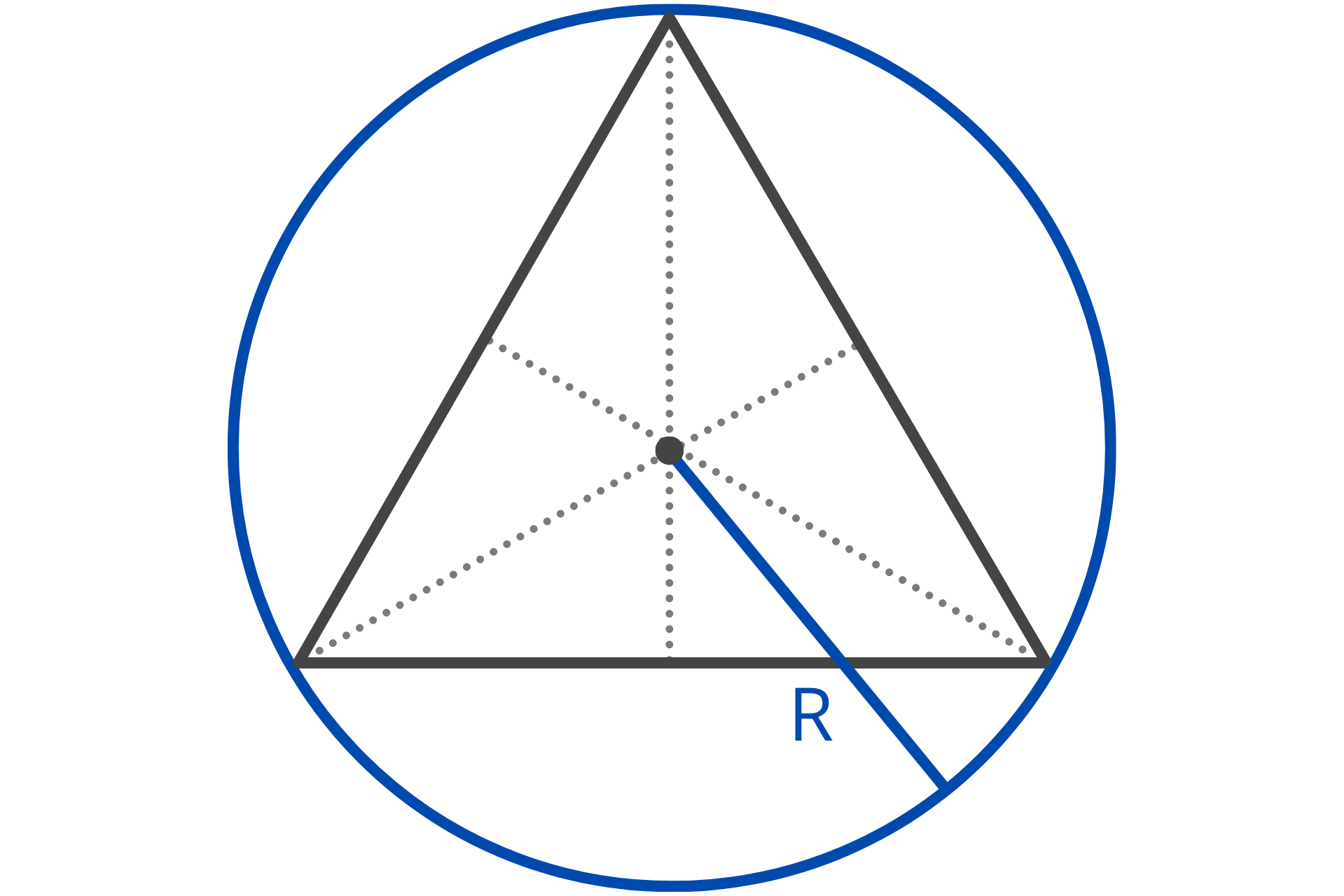# Triangle Calculator

Use our triangle calculator to solve the angles and sides of a triangle using trigonometry. Enter three known values including at least one side to calculate the remaining properties of a triangle.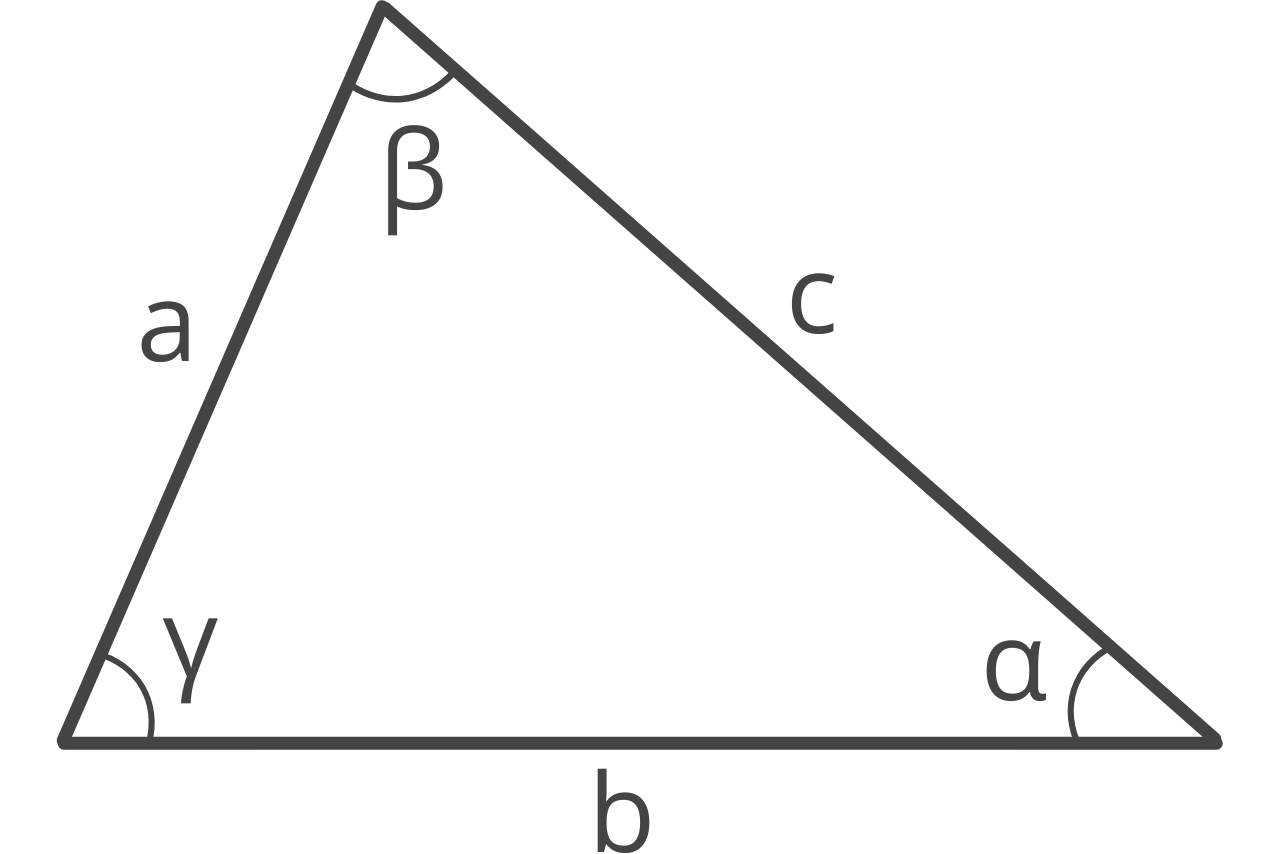## Results:

side a = 10 15 20 28.955 0.50536 Result Unit ° rad 46.5675 0.81276 Result Unit ° rad 104.478 1.8235 Result Unit ° rad 72.6184 45 22.5 14.5237 9.6825 7.2618 16.9558 13.9194 7.9057 3.2275 10.328

### Steps to Solve

Use the Law of Cosines to solve the angles α, β, γ:

\alpha = cos^{-1} \left [ \frac{b^{2} + c^{2} - a^{2}}{2bc} \right ]
\alpha = 28.955\degree
\beta = cos^{-1} \left [ \frac{a^{2} + c^{2} - b^{2}}{2ac} \right ]
\beta = 46.5675\degree
\gamma = cos^{-1} \left [ \frac{a^{2} + b^{2} - c^{2}}{2ab} \right ]
\gamma = 104.478\degree

Solve the perimeter p and semiperimeter s:

p=a+b+c=45
s=\frac{p}{2}=22.5

Use Heron's formula to find the area A:

A=\sqrt{s(s-a)(s-b)(s-c)}
A=72.6184

Solve the height h of each side:

h_{a}=\frac{2A}{a}=14.5237
h_{b}=\frac{2A}{b}=9.6825
h_{c}=\frac{2A}{c}=7.2618

Solve the median m of each side:

m_{a}=\sqrt{(a/2)^{2} + c^{2} - ac \cdot cos(\beta)}
m_{a}=16.9558
m_{b}=\sqrt{(b/2)^{2} + a^{2} - ab \cdot cos(\gamma)}
m_{b}=13.9194
m_{c}=\sqrt{(c/2)^{2} + b^{2} - ab \cdot cos(\alpha)}
m_{c}=7.9057

r=\frac{A}{s}=3.2275
R=\frac{a}{2sin(\alpha)}=10.328
Learn how we calculated this below

## How to Calculate a Triangle’s Missing Sides and Angles

Triangles are polygons with three sides and three interior angles between them. There are several types of triangles, including isosceles, equilateral, scalene, obtuse, acute, and right triangles.

Using special rules such as the Pythagorean theorem, Law of Sines, and Law of Cosines, it’s possible to calculate any angle or side of a triangle if you know the length of at least one side and two remaining side lengths of angles.

### How to Use the Pythagorean Theorem

The Pythagorean theorem states that for a right triangle, the length of the hypotenuse squared is equal to the sum of the squares of each leg. For a right triangle with sides a and b, and a hypotenuse c, the Pythagorean theorem states:

a² + b² = c²

So, if you know the length of any two sides of a right triangle, you can calculate the length of the third side using this formula.

For example, let’s calculate the length of the hypotenuse for a right triangle having sides equal to 5 and 7.

5² + 7² = c²
25 + 49 = c²
74 = c²
√74 = c
8.6 = c

### How to Use the Law of Sines

The Law of Sines or the Sine Rule states that in any oblique triangle, the ratio of a side length to the sine of its opposite angle is constant. The Law of Sines states:

a / sin(α) = b / sin(β) = c / sin(γ)

Where:
a, b, and c are the lengths of the triangle’s sides
α, β, and γ are the angles opposite to sides a, b, and c.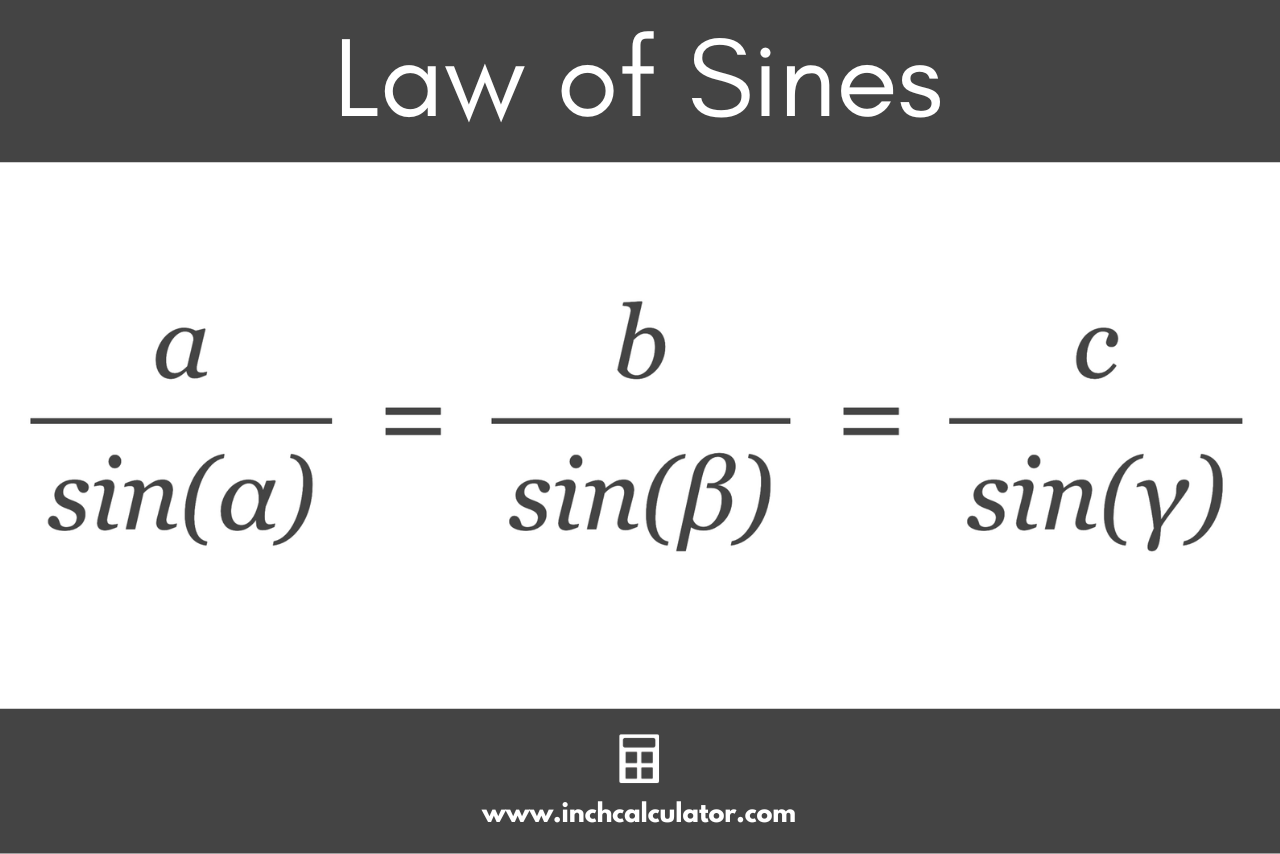You can use this expression to calculate a side length or angle in a triangle if you know the opposite angle and another angle and side length.

For example, let’s calculate the length of side b for a triangle having an angle &alpha equal to 45°, an angle β equal to 75°, and a side a equal to 10.

By substituting the known values into the Law of Sines expression and rearranging, you can solve for side b.

10 / sin(45°) = b / sin(75°)
b = 10 × sin(75°) / sin(45°)
b = 13.66

### How to Use the Law of Cosines

The Law of Cosines or Cosine Rule is a generalization of the Pythagorean theorem formula that relates the lengths of the sides of a triangle to the cosine of its angles. The Law of Cosines states:

a² = b² + c² – 2bc cos(α)
b² = a² + c² – 2ac cos(β)
c² = a² + b² – 2ab cos(γ)

Where:
a, b, and c are the lengths of the triangle’s sides
α, β, and γ are the angles opposite to sides a, b, and c.

By substituting the known values into relevant the Law of Cosines expression and rearranging, you can solve for any remaining side in a triangle.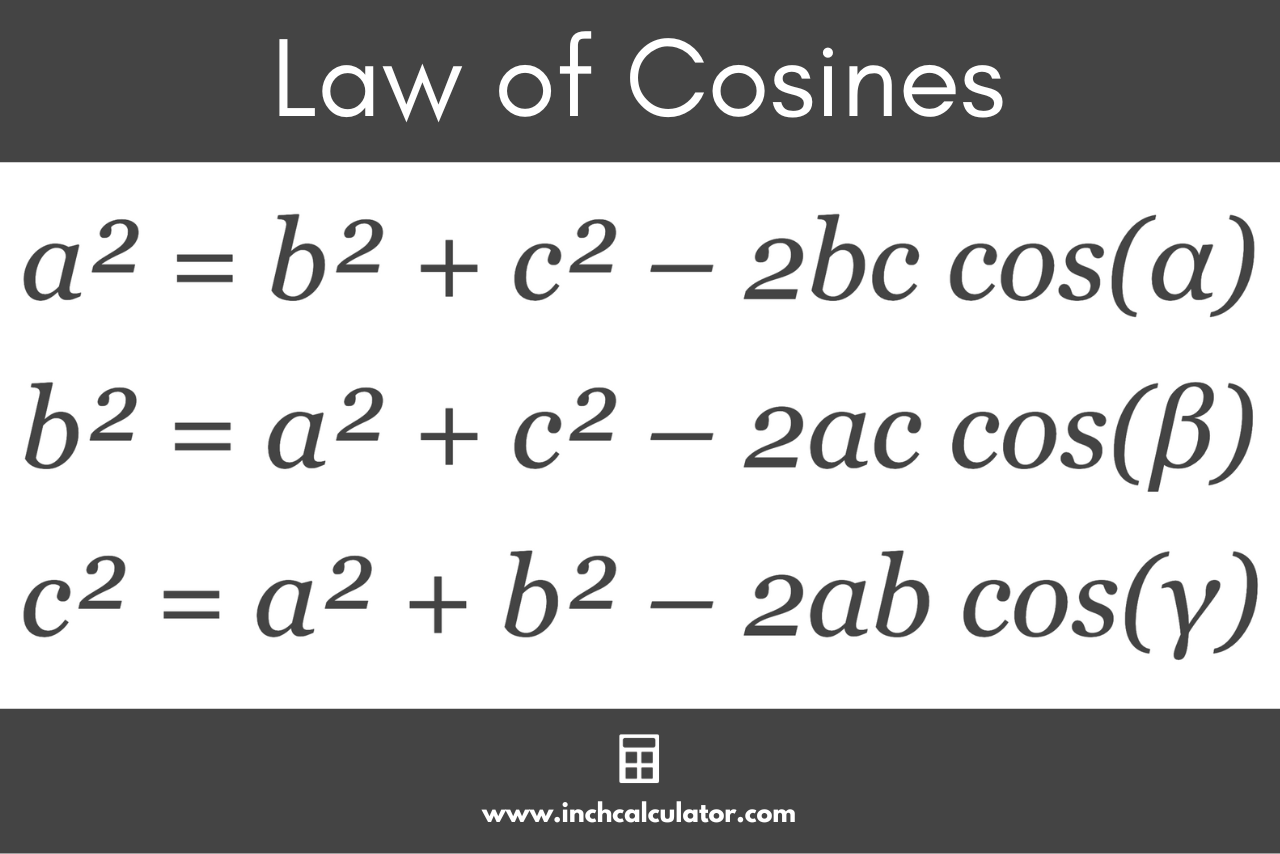For example, let’s calculate the length of side a for a triangle having an angle &alpha equal to 45°, a side b equal to 10, and a side c equal to 14.

By substituting the known values into the Law of Sines expression and rearranging, you can solve for side b.

a² = 10² + 14² – 2 × 10 × 14 cos(45°)
a² = 100 + 196 – 280 × 0.707
a² = 296 – 197.99
a² = 98.01
a = √98.01
a = 9.9

## How to Calculate the Area of a Triangle

You can calculate the area of a triangle using several formulas. The base and height method is the most commonly used. The base and height area formula states:

area = 1 / 2bh

The triangle area is equal to 1/2 times base b times height h.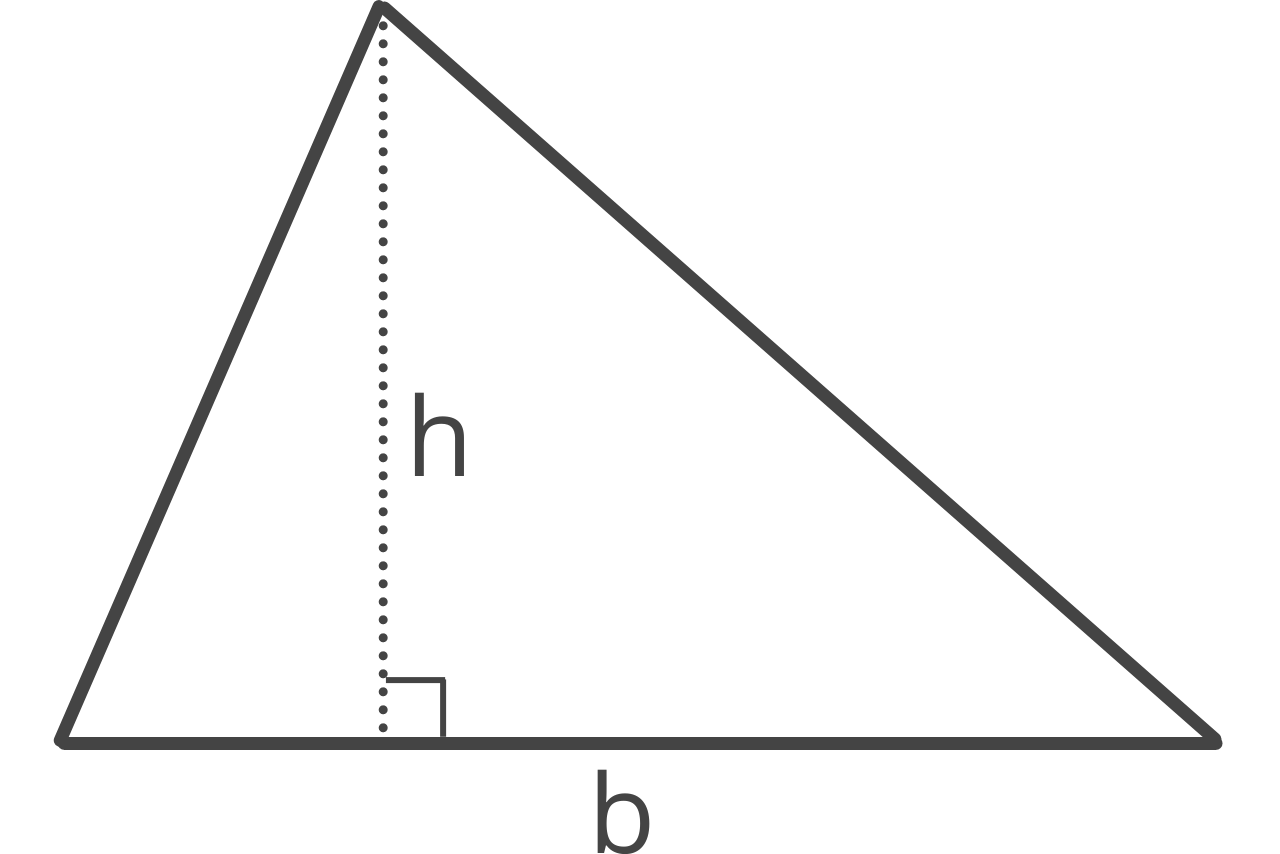You can also use the three sides method (SSS or side-side-side), which is also known as Heron’s formula. Heron’s formula states:

s = a + b + c / 2
area = s(s – a)(s – b)(s – c)

## How to Calculate the Height of a Triangle

You can calculate the height, or altitude, or a triangle using a simple formula using the length of the base and the area.

h = 2A / b

The height of a triangle h is equal to 2 times the area A divided by the length of base b.

The inradius is the radius of the inscribed circle, which is a circle that is tangent to all three sides of the triangle. In other words, it is the largest circle that can fit inside the triangle.

You can use the following formula to calculate the inradius of a triangle.

r = A / s

The inradius r is equal to the area A divided by the semiperimeter s. The semiperimeter is equal to half the perimeter.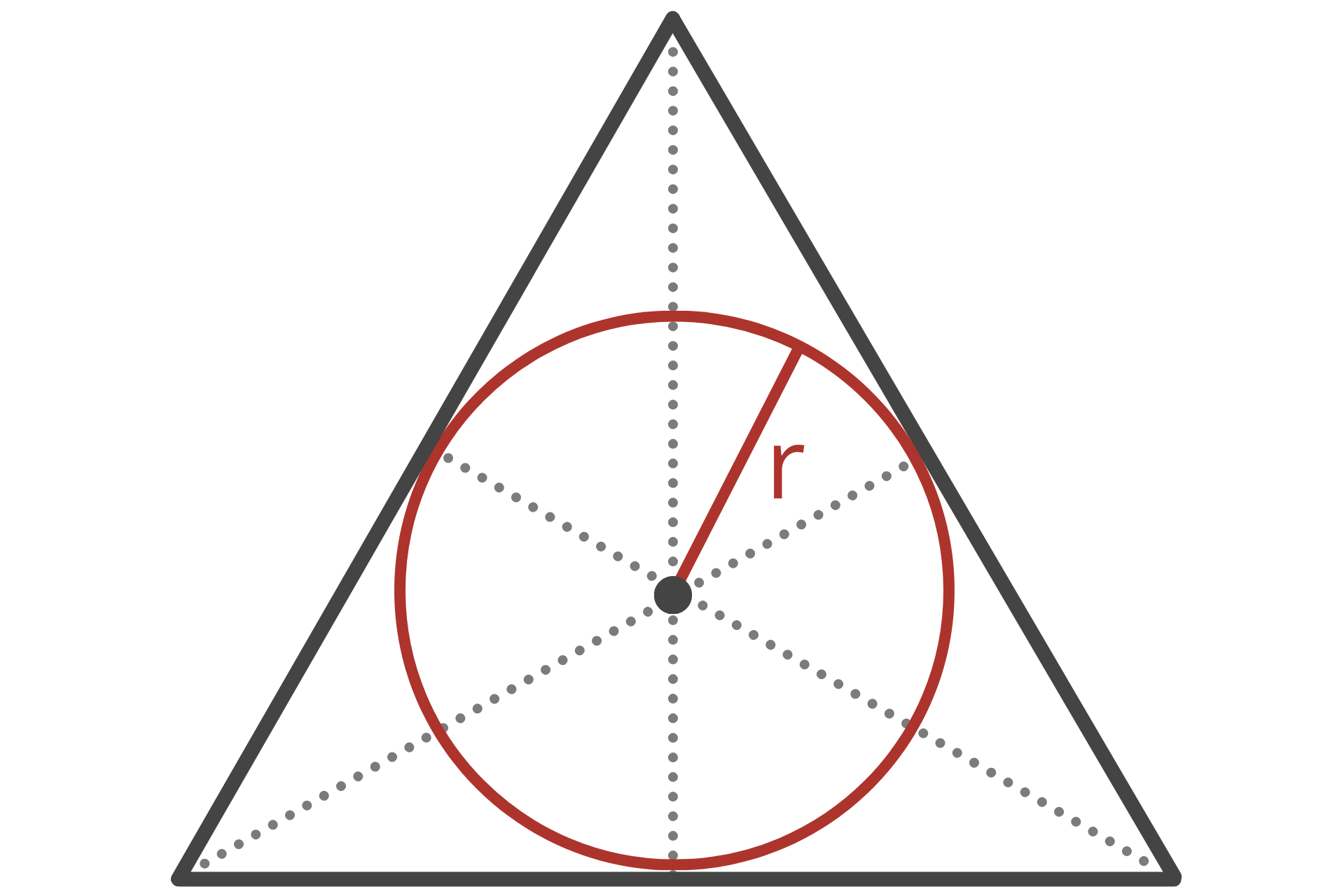The circumradius is the radius of the circumscribed circle, which is the circle that passes through all three vertices of the triangle. In other words, it is the smallest circle that the triangle can fit inside of.

You can use the following formula to calculate the circumradius of a triangle.

R = a / 2sin(α)

The circumradius R is equal to the length of side a divided by 2 times the sine of the opposing angle α.